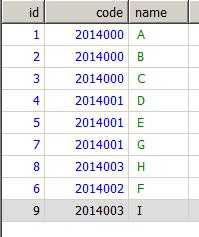﻿ mysql修改问题 | Code Bye

# mysql修改问题

7年前 (2016-09-18) 934次浏览update t a set a.name =(select b.name from t b where b.code=”2014001″ )  where a.code=”2014000″;

10

```update t as c inner join  (select   @ID1:=@ID1+1 as Num,a.ID from t as a,(select @ID1:=0) as Num where a.code="2014000") as a on c.id=a.id
inner join (select   @ID2:=@ID2+1 as Num,a.name from t as a,(select @ID2:=0) as Num where b.code="2014001") as b on  a.Num=b.Num
set c.name=b.name;
```

10

@中国风  题主可以结贴了  高手的sql 中 b.code=”2014001″ 笔误了  改成 a.code=”2014001″  就好了

CodeBye 版权所有丨如未注明 , 均为原创丨本网站采用BY-NC-SA协议进行授权 , 转载请注明mysql修改问题

[1034331897@qq.com]